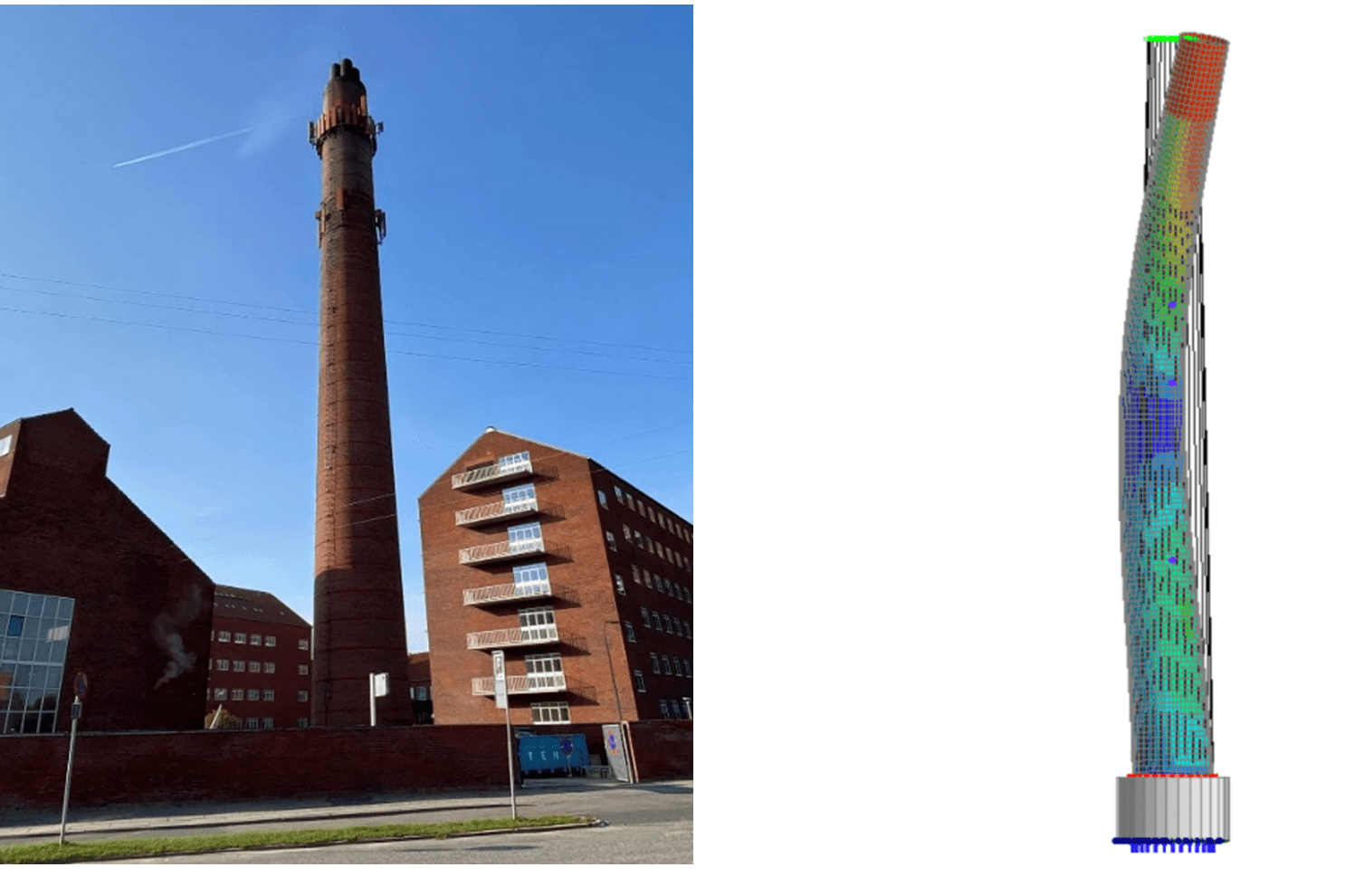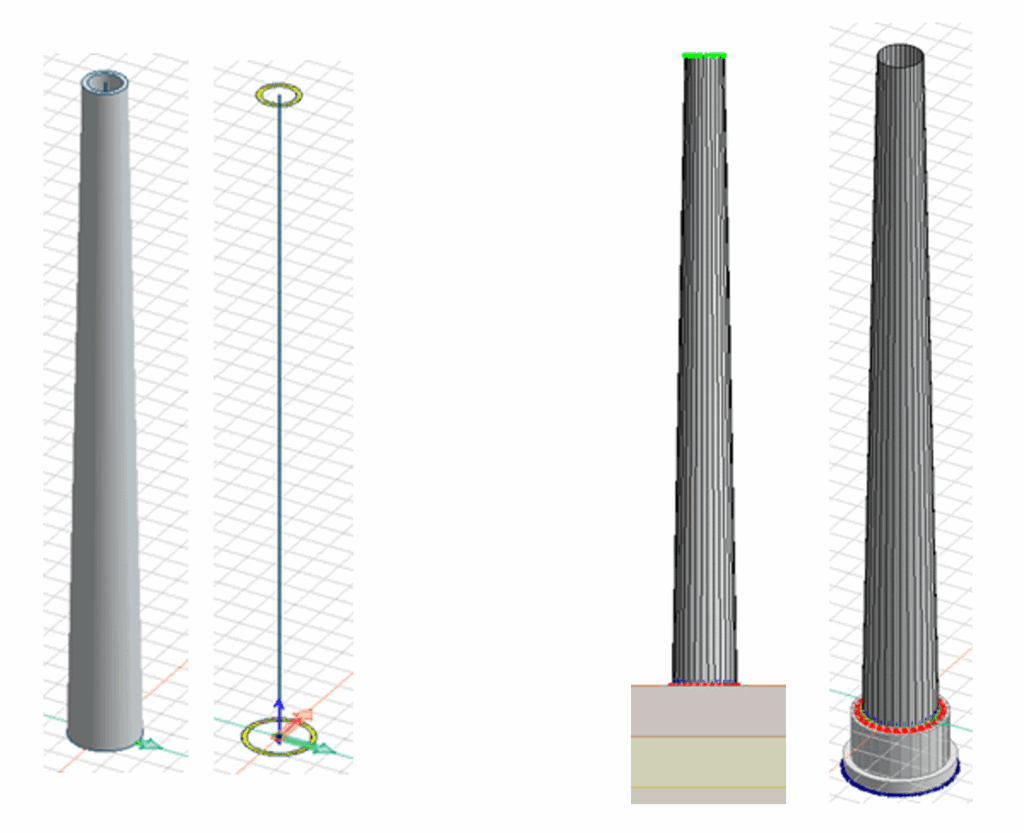Finite element analysis (FEA) is a powerful tool that can be used to simulate the behavior of structures under different loads and conditions. In a recent study, a group of students used FEM-Design, to calculate the eigenfrequencies of a large chimney with the objective of isolating the stiffness of the masonry as the only variable. By doing so, they were able to find the stiffness resulting in the corresponding eigenfrequencies.The students first obtained real-world measurements of the chimney’s eigenfrequencies using a technique called modal testing. Hereafter the students created a detailed 3D model of the chimney using FEM-Design. They then used the software to perform a modal analysis, which is a type of FEA that calculates the natural frequencies and modes of vibration of a structure.

The students found that the eigenfrequencies calculated by FEM-Design were in good agreement with the measurements. They also noted that the accuracy of the results was largely dependent on the quality of the input data and the level of detail included in the model. By taking care to ensure that the model was as accurate as possible and by carefully validating the results, the students were able to demonstrate the effectiveness of FEM-Design in analyzing the behavior of large chimneys and isolating the stiffness of the masonry as the only variable. A stiffness they concluded was between 4600 – 5800 MPa. The variation comes primarily from uncertainty about the density. For a density of 1700 kg/m3 the lowest numerical deviation was found at 5300 MPa.

Overall, this study showed that FEM-Design is a powerful tool for simulating the behavior of structures, and that it can be used to accurately predict the eigenfrequencies of large chimneys when the input data is accurate, and the model is detailed enough. It also highlights the importance of validation of the results using real-world measurements and the importance of isolating the stiffness of the masonry as the only variable in the analysis.

Can you describe the process you used to create the 3D model of the chimney in FEM-Design? What were some of the challenges you faced and how did you overcome them?

The modeling of the chimney is divided into several versions to better determine unknown variables as the modeling becomes more complex.

### SIMPLE MODEL

In the simple model, the chimney is modeled as a fixed column, whereby the stiffness and natural frequencies can be determined based on the material parameters of the masonry. The model provides a clue to determining the final natural frequencies and the stiffness of the chimney in a more complex version.
COMPLEX MODEL

To make the model more realistic, the chimney is modeled with 30 fictitious shells, which makes it easier to adjust the variables behind the results. External loads from antennas and chimneys are also added to the model. Finally, the stair foundation is modeled to determine the support conditions based on FEM-Design’s two calculation methods: Stiffness points and solid Soil.

The stiffness of one fictitious shell is determined from a stiffness matrix, where both the modulus of elasticity and Poisson’s number are included.How closely did the eigenfrequencies calculated by FEM-Design match the real-world measurements? Was there a significant difference, and if so, why do you think that was the case?

The table below shows the average natural frequencies determined by experiments and the natural frequencies determined by FEA. The largest deviation is 1.64% and the total numerical deviation is 3.86%.

Modalshape Data processing FEM-model Deviation
1 0.69 Hz 0.70 Hz 1.48 %
2 3.23 Hz 3.17 Hz -1.64 %
3 7.25 Hz 7.19 Hz 0.74 %Were there any limitations or limitations of using FEM-Design in your project? For example, did it struggle with certain types of loads or boundary conditions?

The modeling was relatively painless. You just must make sure that the fictitious shells border each other. If this is not the case, it will cause problems in the analysis.

For limitations in relation to the calculation of the soil’s stiffness, we experienced that:

1) FEM-Design can only calculate the vertical support stiffness, after which you must manually adjust a factor that determines the horizontal stiffness. (Only applicable to the method with stiffness points)
2) If you do not use 3D soil, and ensure connection between the soil and the foundation, it will require manual modeling work and stiffness calculations to simulate a correct horizontal support of the soil and its resistance to rotation

This was primarily since the natural frequencies in the program came closer to the experimental natural frequencies if the chimney behaved as very/fully pinned support conditions.

Can you talk about the importance of validation and how did you validate your results?

The validation of the results is important to clarify whether the model is correct. Below are various ways in which this is done:

The model’s modal shapes are verified by comparing the found horizontal deformations in FEM-Design with the experimental data. This is done through MAC values (Modal Assurance Criterion), and based on these we can say that the FEM model simulates reality to an acceptable degree

The modulus of elasticity, which partly determines the stiffness of a fictitious shell, has been compared, among other things, with an experiment by SBi carried out in 1992. The modulus of elasticity found in the experiment based on the applicable type of mortar and the compressive strength of the brick is close to the chosen modulus of elasticity in FEM-Design.

Do you think that this kind of simulation can be used in the industry?

FEM-Design has proven to be a useful tool for determining both the chimney’s modulus of elasticity and natural frequencies. Based on experience gained through the project, we have the following comments

• If you want to come up with a precise estimate of a structure’s natural frequencies, then it is necessary to model with a high degree of detail, which is very time-consuming.
• If you simply want the modulus of elasticity of the construction, it suggests that you can settle for a simple model, as the value will thus be on the safe side.

Whether the above conclusions apply to all constructions is difficult to judge from one project, but one thing is clear: FEM-Design is an excellent tool for just this kind of simulation.

The project was done by students at AU Engineering (Arhus University).